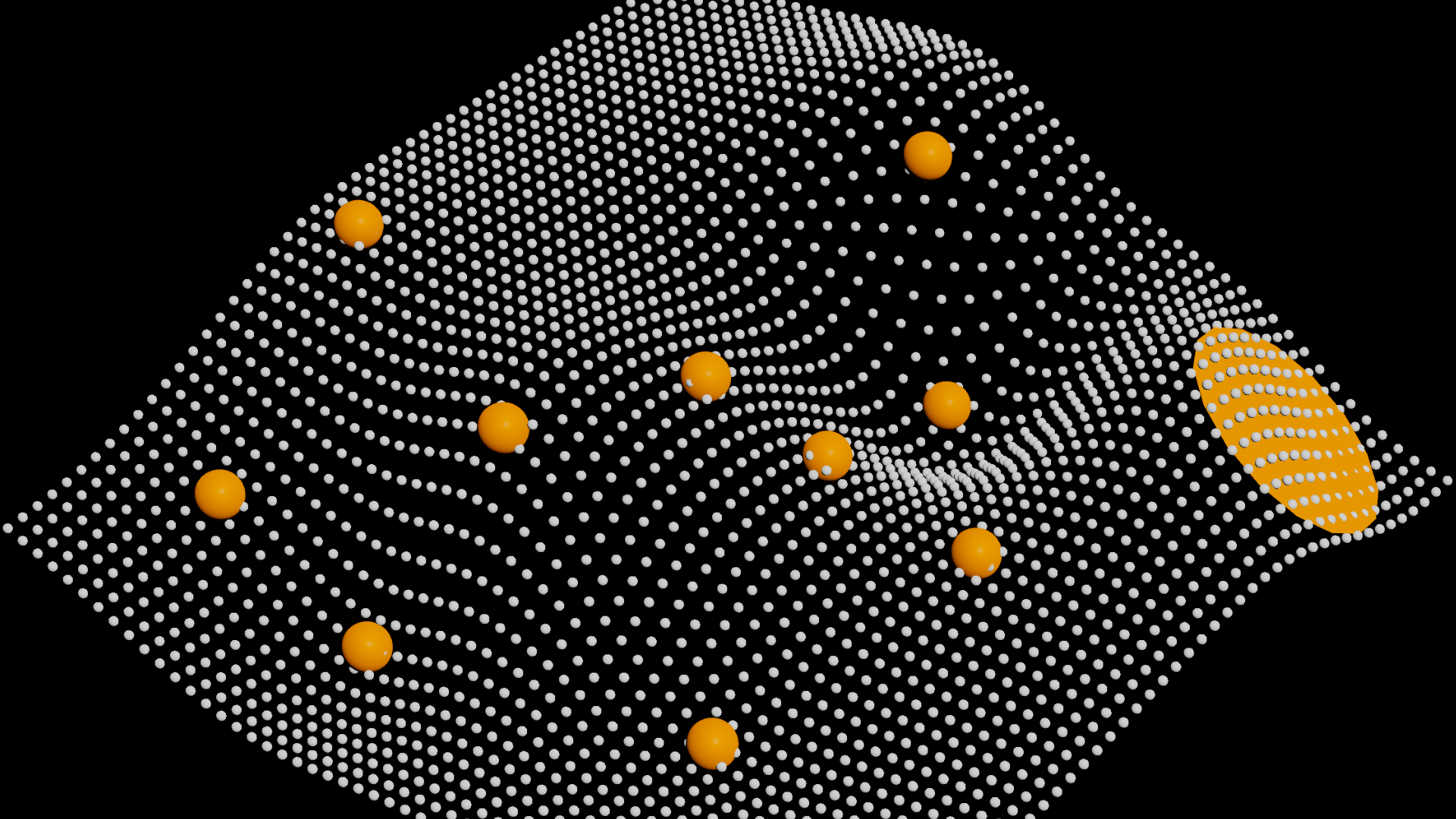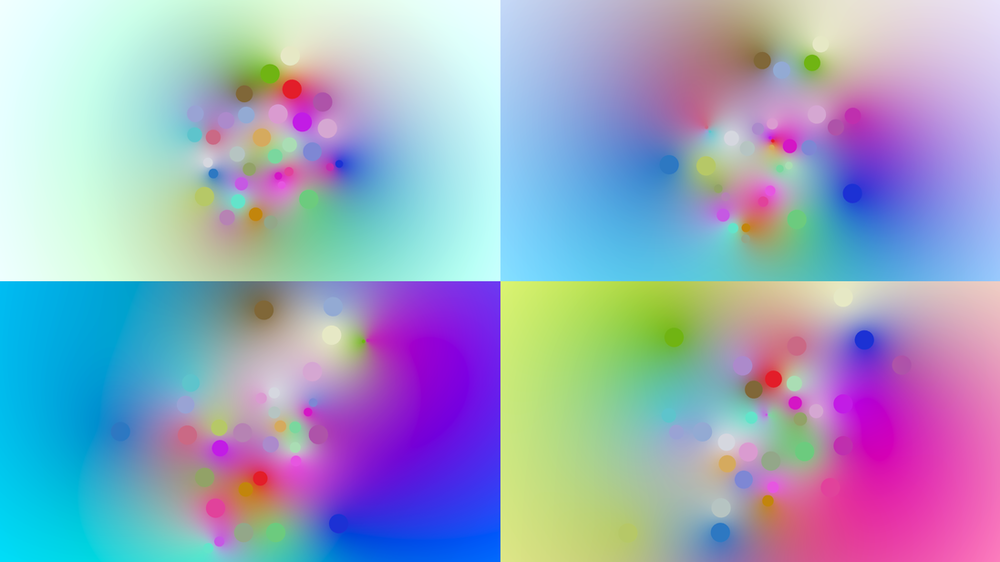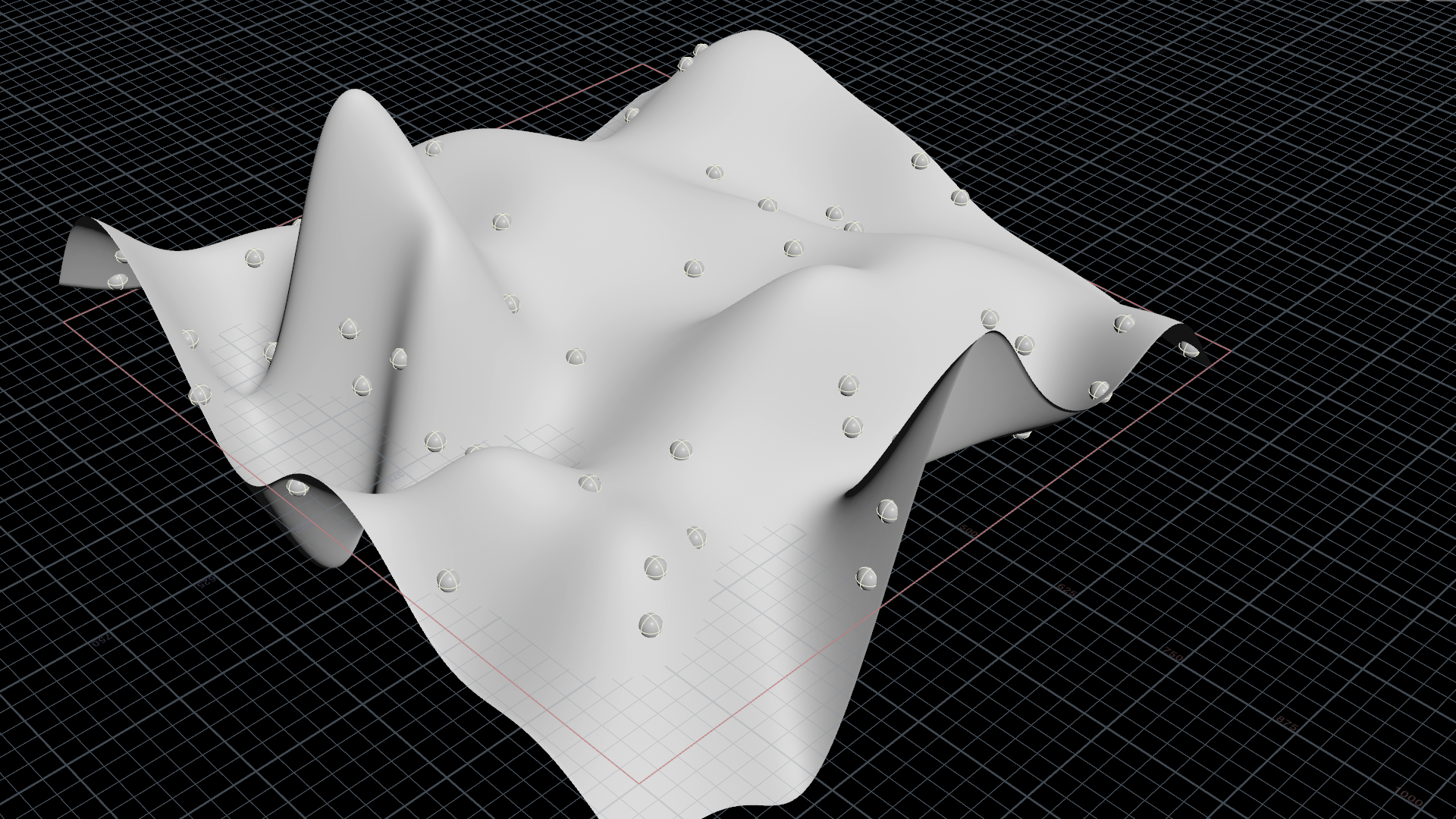# Making a grid flow through guide points

calendar_today

19.06.2022

label

Modeling, Environment, Python

mouse

Houdini 19.0

### 1 Introduction

Making a grid flow through guide points using multivariate interpolation with the Python module Numpy / Scipy.

### 2 Initialization

The `f@height` attribute is going to be interpolated based on common X and Z positions between the grid and the guide points.

``````// Grid
f@px = v@P.x;
f@pz = v@P.z;

f@height = 0.0;``````
``````// Guide points
f@px = v@P.x;
f@pz = v@P.z;

f@height = v@P.y;``````

### 3 Interpolation

Python's NumPy/SciPy library offers multivariate interpolation. It's making sure the grid will always flow smoothly through our guide points even if they are positioned irregularily.

There are various interpolation methodes provided: multiquadric, inverse multiquadric, gaussian, linear, cubic, quintic and thinplate.

``````import numpy as np
from scipy.interpolate import griddata
import scipy.interpolate as interp

node = hou.pwd()
geo1 = node.geometry()
inputs = node.inputs()
geo2 = inputs.geometry()

method_nr = node.evalParm('method')
method_str = method_names[method_nr]

grid_x = np.array(geo1.pointFloatAttribValues('px'))
grid_z = np.array(geo1.pointFloatAttribValues('pz'))
pos_x = np.array(geo2.pointFloatAttribValues('px'))
pos_z = np.array(geo2.pointFloatAttribValues('pz'))
height = np.array(geo2.pointFloatAttribValues('height'))

rbf_height = interp.Rbf(pos_x, pos_z, height, function=method_str)
smooth_rbf_height = rbf_height(grid_x, grid_z)

geo1.setPointFloatAttribValuesFromString("height", smooth_rbf_height.astype(np.float32))``````

### 4 Application

``v@P.y = f@height;``

### 5 ConclusionThe radial basis function (RBF) in Scipy.interpolate does an excellent job at interpolating between irregular points. RBF looks smoother than what we´d get from the Attribute Transfer SOP, too.

### 6 ContributionAdrian Pan contributed a corresponding example file that works directly with heightfields.